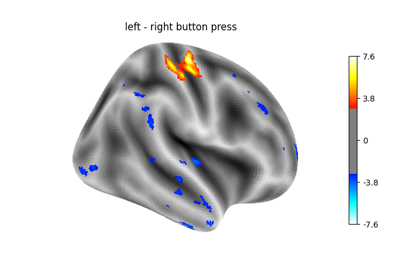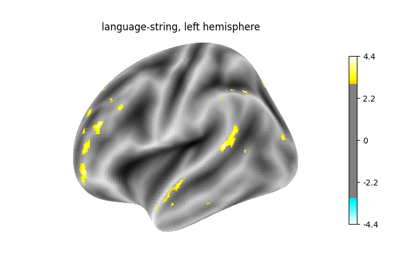Note

This page is a reference documentation. It only explains the function signature, and not how to use it. Please refer to the user guide for the big picture.

3.7.3. nistats.first_level_model.run_glm¶

nistats.first_level_model.run_glm(Y, X, noise_model='ar1', bins=100, n_jobs=1, verbose=0)

GLM fit for an fMRI data matrix

Parameters
Yarray of shape (n_time_points, n_voxels)

The fMRI data.

Xarray of shape (n_time_points, n_regressors)

The design matrix.

noise_model{‘ar1’, ‘ols’}, optional

The temporal variance model. Defaults to ‘ar1’.

binsint, optional

Maximum number of discrete bins for the AR(1) coef histogram.

n_jobsint, optional

The number of CPUs to use to do the computation. -1 means ‘all CPUs’.

verboseint, optional

The verbosity level. Defaut is 0

Returns
labelsarray of shape (n_voxels,),

A map of values on voxels used to identify the corresponding model.

resultsdict,

Keys correspond to the different labels values values are RegressionResults instances corresponding to the voxels.

3.7.3.1. Examples using nistats.first_level_model.run_glm¶